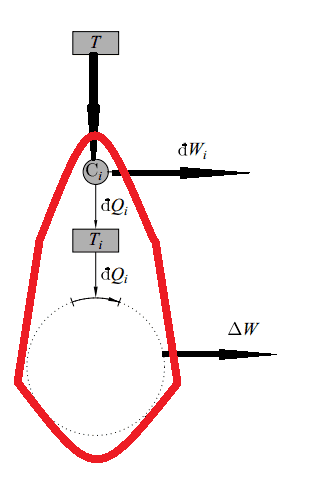# Doubt regarding proof of Clausius Inequality.

• I
I have attached two images from my textbook one of which is a diagram and the other a paragraph with which I am having problems. The last sentence mentions that due to violation of 2nd law we cannot convert all the heat to work in this thermodynamic cycle. However what is preventing the carnot cycles from doing work dW_i ? Shouldn't ΔW be the only work that is impossible to achieve by the machine? Why take ∑dW_i + ΔW <=0 and not just ΔW<=0?

I would also like to know that since at the end of one cycle reservoir the system returns to it's initial state and T loses some heat and if total work performed by that system is zero(for reversible case) then where does the heat go?

#### Attachments

Firstly I am not understanding your difficulty. But here I raise two points of views:
1. There cannot be any proof of Clausius inequality as it is the fundamental axiom in the theory of thermodynamics. One can only relate it to other popular statements of laws of thermodynamics.

2.The ideal reservoirs returning to its original state is also not true in the strictest possible sense because we can go on depositing heat or extracting heat from it and it remains in the same state, one does not know what does it mean totally but only one aspect we know its temperature does not change because of assumed infinite thermal capacity. That is why these heat transfers are considered reversible.

Shouldn't ΔW be the only work that is impossible to achieve by the machine? Why take ∑dW_i + ΔW <=0 and not just ΔW<=0?

No. The system undergoing a thermodynamic cycle is the Carnot cycle plus the other cycle. This is the system that appears to take heat from one reservoir and get work out without rejecting heat to another reservoir. Since for any thermodynamic cycle that is connected to only one reservoir the cyclical work must be less than or equal to zero, it must be so with this cycle too. The cyclical work involved for this cycle is the sum of the individual works outputs from anywhere in the system.

I would also like to know that since at the end of one cycle reservoir the system returns to it's initial state and T loses some heat and if total work performed by that system is zero(for reversible case) then where does the heat go?

If the net work performed by that system is zero, then what is the net heat transfer? Think about it in terms of the first law.

No. The system undergoing a thermodynamic cycle is the Carnot cycle plus the other cycle. This is the system that appears to take heat from one reservoir and get work out without rejecting heat to another reservoir. Since for any thermodynamic cycle that is connected to only one reservoir the cyclical work must be less than or equal to zero, it must be so with this cycle too. The cyclical work involved for this cycle is the sum of the individual works outputs from anywhere in the system.

If the net work performed by that system is zero, then what is the net heat transfer? Think about it in terms of the first law.
But the carnot engine which produces dW_i work is connected to 2 reservoirs. How would this work be negative or zero?

stevendaryl
Staff Emeritus
But the carnot engine which produces dW_i work is connected to 2 reservoirs. How would this work be negative or zero?

Was there more text defining what the quantities $\Delta W$ and $dW_i$ mean?

In the simplest ideal Carnot cycle, there are 4 steps:
1. Isothermal expansion: Put a quantity of gas into a hot reservoir (temperature $T_h$) and let it expand.
2. Isentropic expansion: Take it out of the reservoir and let it expand some more, extracting work from the gas. During this expansion, the temperature drops to $T_c$.
3. Isothermal contraction: Put the gas into a cold reservoir (temperature $T_c$) and let it contract.
4. Isentropic contraction: Take it out of the reservoir and force it to contract some more, putting work into the gas.
In steps 1 and 2, the gas is doing work, and in steps 3 and 4, work is being done on the gas (which is considered to be negative work done by the gas).

The work done by a gas is $P dV$. If the gas is expanding, then it is doing work, and this is a positive number. If the gas is contracting, then work is being done on the gas, and this quantity is a negative number.

But the carnot engine which produces dW_i work is connected to 2 reservoirs. How would this work be negative or zero?

It is not the Carnot engine that is considered in the equation you are asking about. It is the combined system, shown in red in the image below. For this system, would you agree that ##\Delta W+\Sigma dW_i \leq 0##? That is all that they are saying. Once you see this, you should be able to see that ##\Delta W \leq -\Sigma dW_i##.